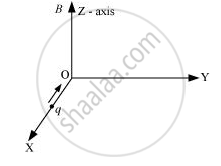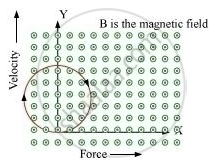Share

# A Charge ‘Q’ Moving Along the X- Axis with a Velocity → V is Subjected to a Uniform Magnetic Field B Along the Z-axis as It Crosses the Origin O. - Physics

#### Question

A charge ‘q’ moving along the X- axis with a velocity  vecv is subjected to a uniform magnetic field B along the Z-axis as it crosses the origin O.(i) Trace its trajectory.

(ii) Does the charge gain kinetic energy as it enters the magnetic field? Justify your answer.

#### Solution

(i)

The direction of magnetic field is along the negative X-direction. Hence, the magnetic force will act in such a way that this particle describes a circular motion as shown below.(ii) No, the charge does not gain kinetic energy because the force and velocity are perpendicular to each other.

W = vecF.vecv =Fvcostheta

theta = 90°

Thus, force does not bring out any change in the velocity.

Is there an error in this question or solution?